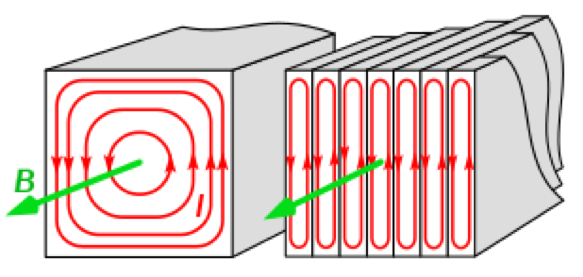# Limitations of Ideal Transformers and Improving Transformer Efficiency

This is part of the HSC Physics course under the topic Electromagnetic Induction.

### HSC Physics Syllabus

• evaluate qualitatively the limitations of the ideal transformer model and the strategies used to improve efficiency, including but not limited to:
- resistive heat production and eddy currents

### Improving Transformer Efficiency

This video will qualitatively evaluate the limitations of the ideal transformer model. It will also explain the strategies used to improve transformer efficiency.

### What is Transformer Efficiency?

Transformer efficiency is defined by the proportion of electrical energy in the primary coil that is transferred to the secondary coil. Ideal transformers have 100% efficiency, which means no energy is transformed to other forms and transferred elsewhere. However, ideal transformers do not exist due to the following limitations.

### Problem 1: Incomplete flux linkage

The relationship between coil turn and voltage heavily assumes that all the magnetic flux in the primary coils is received by the secondary coils. However, in a realistic transformer, some flux is not transmitted through iron core and instead escapes into the air. This limits the efficiency of the transformer.

Solution: As discussed before, using an iron core magnetically links the two coils and helps decrease leakage flux. However, leakage flux can be further decreased by decreasing the distance between the two coils. Generally, this is achieved by coiling the secondary coils over the primary coils around the same limb. The coils are then separated by an insulting layer.

### Problem 2: Resistive heat production due to eddy currents

Since the iron core magnetically links the two coils, it itself also experiences the change in flux caused by the primary coil. The iron core thus experiences an induced emf and, being a closed conductor, will have thus eddy currents flowing in it.

Using right-hand grip rule, the direction of these eddy currents circulate in a plane perpendicular to the plane of the primary coils. These eddy currents lead to resistive heating according to P_(loss) = I^(2)R. As such, energy will be lost as heat

Solution: Laminate the iron core. That is, the iron core is cut into thin slices, with insulating layers inserted between each slice. The place of the slices must be perpendicular to the plane of the primary coils. This orientation best limits the path of the eddy currents, causing them to occur to a lesser extent and thus decreases power loss.### Problem 3: Resistive heat production in coil windings

However, resistive heating also occurs in the primary and secondary coils due to the coils' electrical resistance.

Solution: Resistive heat production can be minimised by reducing the coils' electrical resistance. This can be done by using coils made from materials that have a lower resistance or by increasing the thickness (cross-sectional area, A) of the coils. This causes the resistance of the coils to decrease, according to:

$$R=\frac{\rho l}{A}$$

If R decreases, then P_(loss)=I^(2)R will correspondingly decrease as well.